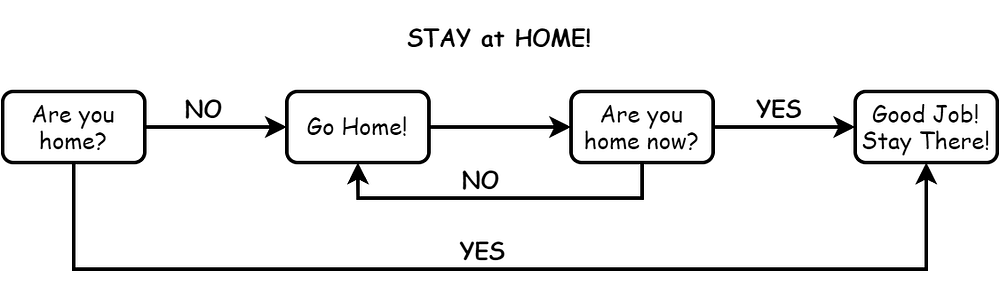# Regression Trees from Scratch in 30 lines of Python

Original article was published on Artificial Intelligence on Medium# Introduction

Flowcharts are used to articulate decision-making processes through a visual medium. Their design requires a complete understanding of the whole system and, thus, human expertise. The question is: “Can we automatically create flowcharts to make their design faster, cheaper, and more scalable with respect to the complexity of the process?” and the answer is decision trees!

Decision trees can automatically deduce rules that best express the inner-workings of decision-making. When trained on a labeled dataset, decision trees learn a tree of rules (i.e. a flowchart) and follow this tree to decide on the output of any given input. Their simplicity and high-interpretability make them a great asset to have in your ML toolbox.

In this story, we describe the regression trees — decision trees with continuous output — and implement code snippets for learning and prediction. We use the Boston dataset to create a use case scenario and learn the rules that define the price of a house. You can find a link to complete code in the references.

# Learning the Rules

We seek a tree of rules, similar to a flowchart, that best explains the relationship between features of a house and its price. Each rule will be a node in this tree and divide houses into disjoint sets, such as houses with two rooms, houses with three rooms and houses with more than three rooms. A rule can be based on multiple features as well, such as houses with two rooms and near the Charles River. Therefore, the space of all possible trees is huge and we need simplifications to computationally tackle the learning.

As the first simplification, we consider only binary rules: rules that divide the houses into two such as “does the house has less than three rooms or not?”. As the second one, we omit the combinations of features since the number of combinations can be huge and consider rules based only on one feature. Under these simplifications, a rule is a “less than relation” with two parts: a feature, such as the number of rooms and the division threshold such as three.

Based on this rule definition, we construct the rule tree by recursively seeking the rules that best divide the data into two.

In other words, we first divide the data into two splits as best as we can and then consider each split separately for the division again. We continue dividing the splits until a pre-defined condition such as maximum depth is satisfied. The constructed tree is only an approximation of the best tree due to simplifications and greedy rule search. Below you can find a Python code that implements the learning.

We implement the splitting procedure as a function and call it with training data (X_train, y_train). The function finds the best rule to divide the training data into two and performs the splitting according to the found rule. It keeps calling itself by using left and right splits as training data until the pre-specified maximum depth is reached or training data is too small to divide. When the stopping condition is met, it stops division and predicts the house prices as the mean price of the training data in the current split.

In the split function, a division rule is defined as a dictionary with keys left, right, feature, and threshold. The best division rule is returned by another function that exhaustively scans possible rules by traversing each feature and threshold in the training set. The thresholds to try out for a feature are determined bt the values the feature takes across the dataset. Here is the code:

The function keeps track of the best rule by measuring the quality of the split proposed by the rule. The quality is measured by a “the lower the better metric” named residual squared sum (RSS) (see the notebook in references for more detail on RSS). Last, the best rule is returned as a dictionary.

# Interpreting the Rules

The learning algorithm automatically chose features and thresholds to create rules that best explains the relationship between the features of a house and its price. Below we visualize the tree of rules learned from the Boston dataset with a maximum depth of 3. We can observe that extracted rules overlap with human intuition. Besides, we can predict the price of a house as easy as tracing a flowchart.